Chen, J., He, G., Wang, X., Wang, J., Yi, J., and Yang, C. (2020). "Theoretical and experimental research on slip and uplift of the timber-concrete composite beam," BioRes. 15(3), 7079-7099.

#### Abstract

Timber-concrete composite beams are a new type of structural element that is environmentally friendly. The structural efficiency of this kind of beam highly depends on the stiffness of the interlayer connection. The structural efficiency of the composite was evaluated by experimental and theoretical investigations performed on the relative horizontal slip and vertical uplift along the interlayer between composite’s timber and concrete slab. Differential equations were established based on a theoretical analysis of combination effects of interlayer slip and vertical uplift, by using deformation theory of elastics. Subsequently, the differential equations were solved and the magnitude of uplift force at the interlayer was obtained. It was concluded that the theoretical calculations were in good agreement with the results of experimentation.

Theoretical and Experimental Research on Slip and Uplift of the Timber-Concrete Composite Beam

Jianying Chen,a Guojing He,a,* Xiaodong (Alice) Wang,b Jiejun Wang,a JinYi,a and Chuanjian Yang a

Timber-concrete composite beams are a new type of structural element that is environmentally friendly. The structural efficiency of this kind of beam highly depends on the stiffness of the interlayer connection. The structural efficiency of the composite was evaluated by experimental and theoretical investigations performed on the relative horizontal slip and vertical uplift along the interlayer between composite’s timber and concrete slab. Differential equations were established based on a theoretical analysis of combination effects of interlayer slip and vertical uplift, by using deformation theory of elastics. Subsequently, the differential equations were solved and the magnitude of uplift force at the interlayer was obtained. It was concluded that the theoretical calculations were in good agreement with the results of experimentation.

Keywords: Timber-concrete composite beam; Interlayer slip; Vertical uplift; bolt; Differential equations

Contact information: a: College of Civil Engineering, Central South University of Forestry and Technology, 498 Shaoshan Road, Changsha, Hunan, 410004 China; b: Department of Wood and Forest Sciences, Pavillon Kruger, Laval University, Quebec, Q. C., Canada G1V 0A6;

* Corresponding author: znlykj498cjy@hotmail.com; cegjhe@hotmail.com; cjy801123@126.com

INTRODUCTION

The timber-concrete composite (TCC) structure is a construction technique in which a timber beam is connected to an upper concrete slab using different types of connectors. The advantages of the different materials properties can be utilized, where bending and tensile forces get primarily resisted by the timber, whereas the compression force is resisted by the concrete topping (Gutkowski et al. 2008). The structural efficiency of a TCC highly depends on the stiffness of the interlayer connection (Yeho et al. 2011), where shear deformation and axial deformation of a flexible shear connector, such as a bolt, leads to an inevitable interlayer slip and vertical uplift between the timber beam and the concrete slab. This action reduces the combination effect and stiffness of the composite-beam section, resulting in decreased bending-loading capacity and increased deformation of the composite beam (Rodrigues et al. 2013). Studies of the interlayer slip, vertical-uplift pattern, and their influence on the ultimate loading capacity and deformation of the composite beam structure are therefore needed.

Previous studies aimed at describing this composite structure have analyzed the relative horizontal slip caused by longitudinal shearing along the interlayer between timber beams and the concrete slab by use of various numerical methods, including finite element methods (Ayoub 2001, 2005; Ranzi et al.2004; Cas et al.2004; EN1995-2 2004; Jorge et al. 2010; Dias et al. 2011, 2012, 2015; Lopes et al. 2012; Martins et al. 2016; Cas et al. 2018). However, investigations of the vertical-uplift force between timber beams and the concrete slab are rare. The finite element model of the composite beam proposed by Gara et al. (2006) was the only model that considered longitudinal slip and vertical uplift. In the British codes (BS5400-5. 2005), the shear resistance of the connector in composite beams and its vertical-uplift force must be computed. However, the method of the calculation is not specified in the code.

The objective of this study was to research the influence on the interface slip and vertical lifting force by changing the shear connection ratios and the arrangement of the bolts. The specific objective was to calculate the vertical-uplift force and develop differential equations of simply supported TCC beams with respect to the interface slip and vertical uplift.

EXPERIMENTAL

Specimens and Material Properties

The experimental tests investigated the influence of different shear connection ratios and arrangement of shear connectors on relative horizontal slip and vertical-uplift force. The bolt connector is assumed to act as a tension member of the vertical-uplift force in the experiment. Additionally, the uplift of the composite beam is assumed to be small, and the axial tensile stress of the bolt cannot reach the tensile yield strength. The strain gauges were attached to each side of the middle section of the bolt rod and perpendicular to the longitudinal direction of the beam. The experimental magnitude of the uplift force on the bolt can be calculated based on the measured strain under load and the bolt properties, as shown in Eq. 1.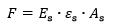(1)

In this equation, F is the vertical-uplift force, is the elastic modulus of the bolt, is the average strain of the two sides of the bolt, and is the cross-sectional area of the bolt.

Five simply supported 2.8 m span TCC beams with bolt connectors were constructed for the experiment. To enhance the overall performance of composite beams, the concrete slabs were configured with longitudinal and horizontal steel bars with yield strength of 330 MPa. The diameter of these steel bars was 8 mm, and the spacing was 100 mm. By changing the shear connection ratios of connection and arrangement of the bolts, this work studied the influence of interface slip and vertical lifting force. The parameters of each specimen are shown in Table 1, and the construction process of the beams is shown in Fig. 1.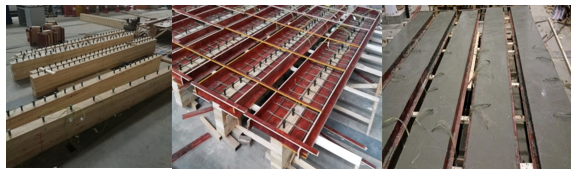Fig. 1. Construction of the TCC

The structural efficiency of a TCC beam highly depends on the number of shear connectors. In this study, the shear connection ratios of composite beams was evaluated in terms of the ratio of the number of shear connectors (n) used in the composite beam, to the number of shear connectors used in the composite beam with a full shear connection (), i.e. (n/nf). The number of shear connectors used in the composite beam with a full shear connection () can be calculated using Eq. 2,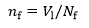(2)

whereis the maximum longitudinal shear force at the interface and Nf is the shear bearing capacity of an individual connector with a theoretical value of 26.6 kN (He et al. 2016).

Table 1. Timber-Concrete Composite Beam Specimen Parameters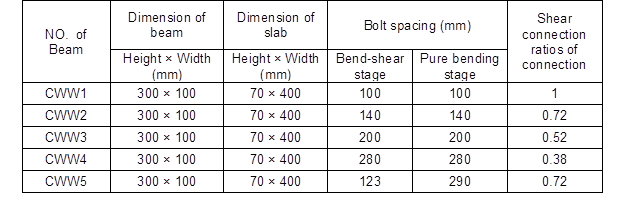Timber

The glued timber blocks used in the tests were made of Xing’an larch wood and joined with polyurethane glue. Six specimens were tested according to the in the test method for tensile strength parallel to grain of wood (GB/T 1938-2009) (Fig. 2), where the tensile strength was found to be 115.9 MPa. According to the method of testing in compressive strength parallel to grain of wood (GB/T 1935-2009), six full scale specimens with dimensions of 30 mm x20 mm x 20 mm were tested (Fig. 3), where the compressive strength was 48.2 MPa. The modulus of elasticity in compression parallel to grain of wood (GB/T 15777-2017) was tested for seven full scale specimens with dimensions of 60 mm × 20 mm × 20 mm were tested (Fig. 4), and the modulus of elasticity was 13.8 GPa.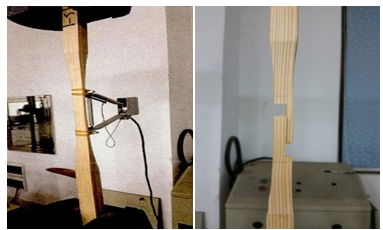Fig. 2. Tensile strength test of timber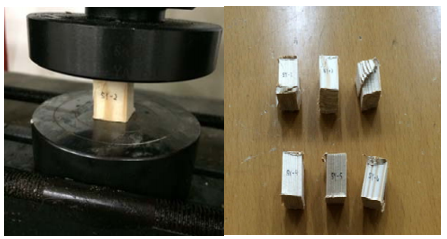Fig. 3. Compressive strength test of timber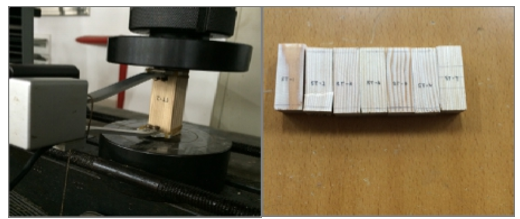Fig. 4. Test for the modulus of elasticity in compression parallel to grain of wood

The density of the timber was determined for six wood pieces with the dimension 20 mm × 20 mm × 20 mm. The mean density of the timber was found to be 552.0 kg/m3.

Concrete

Six concrete blocks with a size of 150 mm×150 mm×150mm were made in the laboratory of Central South University of Forestry and Technology, where also the five TCC slabs were cast. The expected strength class of the concrete was C30. The actual mean strength of the concrete was 31.78 MPa, and the actual mean density of concrete was found to be 2385.0 kg/m(Fig. 5). The elastic modulus of the concrete was 30 GPa.

The high-strength bolts were of diameter 16 mm and length 120 mm, had M-threading and were bought from Suzhou Qiangda Fastener Industry Co., Suzhou, China. The material parameters were provided by the manufacturer, where the bolt tensile strength was 1200 MPa with yield strength of 1080 MPa. The elastic modulus of the bolt was 210 GPa. In this paper, the bolt-penetration depth in the timber was 80 mm.

Base on the following Eq. 3, the horizontal shear stiffness (Kl) of the bolts in the positive moment region was calculated to be 7862 N/mm by using the experimental properties of the concrete and bolts.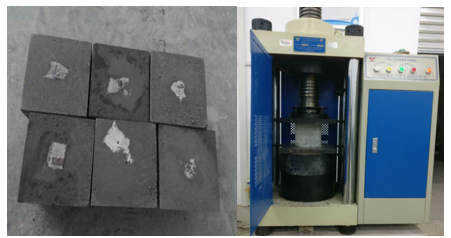Fig. 5. Compressive strength test of concrete

Test Device and Procedure